## The Mathematics of the Giza Site Plan

Foreword

This piece would not have been written if it weren’t for the inspiring work of John Legon on Egypt’s Giza site which you will find at ‘Egyptology and the Giza Pyramids’. Although his important insights have been around for quite some time (1979), they seem to have been pushed to the background. Nevertheless in my view one of his major points to argue for overall Giza site plan dimensions that would be characterized by a length of 1000√3 and a width of 1000√2 expressed in Royal Cubits, which in numbers is 1732RC (907.135m) and 1414RC (740.58m)respectively, still stands, as I will argue here.

The importance for evidence of one mathematical concept is that it again stresses the meaning of the site, that is, to express divine mathematical truth in dimensions of geometry. The pyramid in this concept is not in the first place a -symbolic- tomb, but a temple to the gods, possibly to Thoth, the god of divine knowledge and the keeper of the calendar.

In the following I expand on my own mathematical approach to Giza and I show a different solution for Legon’s  two square roots in a ground plan that is not only based on whole numbers all the way, unlike his, but with striking dimension relations and cross-referencing between the three buildings which show us a work of outstanding mathematical beauty and cosmological depth.
Here is his Site plan measured in Royal Cubits of 0.52375m (remember I use the slightly smaller 0.5236m, which is though immaterial here):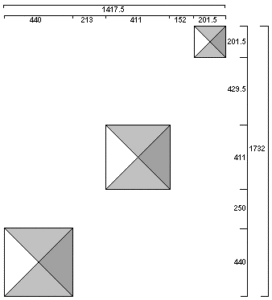Below is the Great Pyramid (Khufu), in the middle the Second Pyramid (Khafre) and above the Third Pyramid (Menkaure), they are all taken to be (near-) squares at the base. North-South is upside down in this pictograph.

The Third is the key

[[May ’18. Recently I have come in contact with John Legon after he commented favourably in a letter on my website. I visited him twice and we have had regular contact ever since. From these exchanges of ideas and insights some new and interesting facts may have come forward. The most important new fact for me is that John calculates that the dressed platform on the east side of the Third Pyramid marks a level where the side of the pyramid is 198RC. Since at the other pyramids the mathematical measure is taken at the platform level, also here this should be done similarly in my view, which then as 198RC confirms my analysis of Menkaure as key mathematical prototype design for the GP and entails a theoretical height of 126RC for the pyramid from the platform level. The number 126 (9×14) is typically in accord with the theoretical height of the Second Pyramid at 274 which taken together results in 400; 280 added gives 680, a number which returns elsewhere (40×17) (Legon: 220+680=900). What we see here as well is the special indication of the length 198RC as we saw this under the top of the Great Pyramid at airshaft exit level. Both subtle architectural pointers say a lot about the importance being given to the ‘traceability’, even visibility, of the underlying mathematics in the design.
According to Legon’s analysis the base measurements of the Third Pyramid expressed in palms are striking because at the level Petrie measured as mathematical level (he even opted for 200RC! ) the square-side could be 202RC (instead of 201.5RC) which is exactly 1414 palms which is 1000 sqrt(2), 1/7 of the site-side, so the diagonal then must be 2000RC, which, halved for the centre, is 1000RC and with the same seked of 5.5   as the GP, this entails a height of 900 palms (ratio 10:9) (67.32m). Legon has noticed this in earlier work. So what we see in this one building is twice the rationalisation of sqrt(2) at different levels. We see it in palms on  a side 1414, a diagonal 2000, halved 1000 and a height of 900palms; in cubits on a side 198, a diagonal 280, halved 140 and a height of 126.
This double confirmation of the cardinal ratios  of the theorem (11:7 & 10:9) and the square root relation in whole cubits as well as in whole palms is not only aesthetically very attractive and harmonious, but also gives strong circumstantial evidence that the square roots play an important (hidden) role in the design, which John’s work highlights.]]

I have argued on this website in a rather different context for the measure of the side of the Third Pyramid (TP) to be taken as 198RC = 103.67m and the height as 126RC= 65.97m (see Orkney and Giza) which makes this Pyramid the mathematical prototype of the Great Pyramid (GP). My argument for the 198RC and 126RC base lengths is foremost mathematical and circumstantial, but also born from the conviction that what we have to do as researchers and scientists in these matters is finding the most logical, simple and reasonable working hypothesis, given the overwhelmingly evident mathematical context and given that we will never know precisely what they had in mind, unless at some point papyrus scrolls are found that give the original outlay of the plan. We don’t have those so we must imagine and reason what they could have had in mind when creating these designs based on their inner logic, the perennial attraction and agony of archaeology.
At least Legon’s insights strongly support the inference that the whole site was a total concept from the beginning, as I have come to think as well. This could very well mean that the positions and dimensions of the three pyramids at Giza were set out with the same unit of length. I emphasize this because I use a slightly different unit of length than is custom, that is 52.36cm instead of Petrie’s 52.375cm, it is though within the margin of error he gives (20.62 in +/- 0.005in, 20.615in = 0.5236 m). (The cubit of the King’s chamber construction is, I think, not the same as the initial one setting the ground plan, many years earlier)

I have written elsewhere that in my view Pharao Sneferu, Khufu’s father, who built the first three ‘true’ pyramids-ever in his lifetime, could have been the great architect of Giza and possibly a mathematician himself. His first work, the pyramid of Meidum, a possible reconstruction of an earlier step-pyramid, has the same ratios as the Great Pyramid, that is, base 275RC with height 175RC = 11 : 7 (440 : 280 = 11 : 7, seked 5.5). I take it that Sneferu’s lineage took it upon themselves to realize his grand mathematical and cosmological design in stone (except for the intermezzo of the short reigning Djedefre, son of Khufu, who broke with the religious tradition and built a pyramid away from Giza and was succeeded by his brother Khafre, the builder of the Second Pyramid, who restored the traditional religion). Even Snefru’s great-grandson  Menkaure, building the much less impressive Third Pyramid, stuck to the original design of his great-grandfather, in our understanding.
Anyway Sneferu was such an impressive personality and successful ruler that he became deified and widely worshipped after his death. His massive ‘state works’ brought great prosperity to Egypt with people employed and skilled on a scale never seen before.

The proof

Let me first give my whole number solution to Legon’s claim of the overall Giza Site dimensions in ratio √2 (1.414 213562…) to √3 (1.732 0508….). I will use Legon’s pictograph and show the three measures I have changed and the consequences.
For the short side I only have to change Legon’s (=F.Petrie’s) 201.5 RC into 198RC to get the perfect 1000√2 = 1414RC because Legon’s anomalous sum of 1417.5 minus 3.5 = 1414  (201.5 – 198 = 3.5), so the correct sum would be 440 GP + 213  + 411 SP + 152 + 198 TP = 1414RC, which by definition is 2000 Remen (the Remen according to Petrie in Nature was the land-measuring rod),  the rest is not contested here, we even see the spacing conspicuously as 213 + 152 = 365, the days of the year.
For the long side we only have to change 201.5RC into 198RC again and 429.5 into 433RC, so both Legon’s broken number data change to whole numbers here, and we get  440 GP + 250 + 411 SP + 433 + 198 TP = 1732, which is the required 1000√3, which is virtually 2450 Remen. (see also 2450 x 2 = 4900 = 70²)
A strong argument for the correctness of 433RC, which appears also at another place (220+213=433), is that 433/250 = 1.732 which is again √3.
What more do we want? Is this not intricate and enigmatic in itself, and full of mathematical meaning?So the question is why do I think the 198RC for the side of the Third Pyramid is what they (or Sneferu) at least had in mind, if not perhaps perfectly executed. The reason is that other values make no mathematical sense, at least not as clear as the 198RC x 198RC I suggest does, which has clear cross-referencing with the Great Pyramid. (the diagonal of 198² = 280.014… )
My mathematical analysis is physically supported by a dressed platform (of 198RC^2 ) at the centre of the east side of the TP indicating the level of the 198RC side length and 126RC height point, as John Legon made me aware of.
It is said that the Third Pyramid was less well built as the others, so it may be slightly sagged here and there, which also might make for the different interpretations and choices the surveyors make in their final verdicts. I hold that the original TP (in design) had a seked like the GP of 5.5 , while others claim on different bases it would be 4:5, which is only a slight difference of 275:280 = 55:56, but unlikely.

This website is about the natural number mathematics of the complementary ratios 7 : 11 : 14 with a π-value of 22/7 (3.142857….) and 9 : 10 : 11 with a π-value of 2800/891 (3.142536….) which taken together produce an irrational Pi: 20√2/9 (3.1426968…, coined Qute here), being the root of a π²- value 800/81 (22/7 x 2800/891) and these differing values then  in relation to circles and squares, as representatives of spheres and horn-tori, represent the basic mathematical dynamics of space, of nature.

The dimensions of the pyramid of Menkaure signifiy the basics of this natural number geometry, brought back to the smallest natural numbers (99, 126, 140, 198, 280), in an elegant structure which is the mathematical key to understanding the dimensions and symbolism of the GP, that is, revealing the radius and circumference of the Earth.
It is quite well-known that the crux and genius of the Great Pyramid design is that its height signifies the radius of a circle of which the circumference is equal to its square base perimeter, like is exemplified in the pictogram below:
(Beware we use here the Pi value 22/7 on which the GP design is based)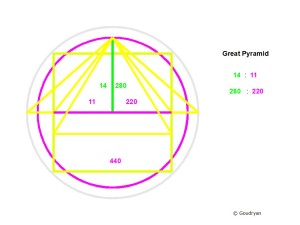When we square a circle by circumference and circumscribe this square with a new circle (grey) we will always see that the radii of the two circles relate as 9:10 (see below) This can only be done by using a Pi value of square root (800/81)= √(8 x (10/9)²) = 2.22222…√2 (3.14269680…)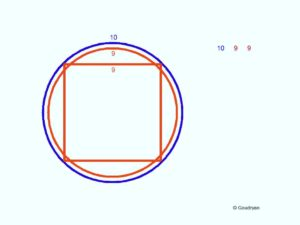Here we see the foundation of the relation between circle and square with equal perimeter. The circles 9 and 10 can be read in many ways because they signify ratios, it means that 9 can be cord length, with 10 as its circle segment, but also that 9 is the radius of circle 9 and 10 the radius of circle 10. What it means is that any circle circumference changed into a square perimeter will have a diagonal which is related to the radius of the original circle as 10 : 9 or 11 : 9.9 or 110:99 or 220/198 etc.  (with official Pi it is 10 : 9.00317…. a trifling difference and irrelevant in resonance)
This way we can find several possibilities of rationalising the square root of 2 in natural numbers, here as 140/99, but also 99/70 or doubled 198/140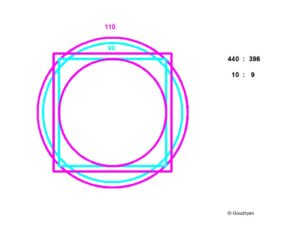In the following diagram we find the ratios that relate to the seked 5.5 (7 : 5.5 = 14 :11) of the GP, that is the height related to the half side, but also the height related to the half diagonal as 9 : 10 which expresses itself in the angle of the arris edge of the Pyramid, when we want to match these two ratios in whole numbers we have to multiply 14 x 9 = 126, where follow a half side of 99 and a half diagonal of 140. From these ratios it emerges that a pyramid height of 126RC corresponds to a base side of 2 x 99 = 198RC, with a diagonal of 2 x 140 = 280RC, which are the supposed mathematical dimensions of the Third Pyramid. So height 126RC = 65.97m, side 198RC = 103.67-70m  and diagonal 280RC = 146.61m, which is the original height of the Great Pyramid, an obvious cross reference.
But that is not all because the 198RC and 126RC refer respectively to the height of the GP from the floor level of the King’s chamber at 82RC (280-82=198) up to the top and secondly to the height of the GP at the air shaft exit level from 154RC (280-154=126) up to the top at 280RC. Also at air shaft exit level the side of the GP is 198RC, which means that the top of the GP from airshaft exit level is identical to the mathematical Third Pyramid (at platform pavement level). The mathematical volume of the Third Pyramid is therefore virtually 1 : 11 (10.97) of the volume of the GP, which means that the top of the GP at air shaft level is 1/10 of the rest of the pyramid down to the pavements.
So the geometry of square root 2 is: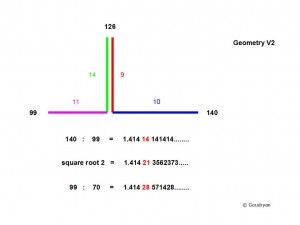As regards the Great Pyramid we can express the height in Remen, is 99/70 x 280 = 396 Remen and the half diagonal as 440 Remen so we find also here the desired ratio  440 : 396 = 10 : 9. The Remen can not be fully employed in the TP, neither the RC in the GP. Another complementary  situation.

All this proves that the Third Pyramid could well be seen as the mathematical key to the Great Pyramid. QED.